# Generating Negative Output Voltage from Positive Input Voltage Using MAX17291 Boost Converter IC with Active Discharge Feature

### 要約

This application note explains how to generate a negative voltage from positive input voltage using MAX17291 for applications, such as LCD displays, gate drivers, etc. It also includes information on implementing the active discharge feature.

#### Introduction

Many applications require the power supply to provide a negative voltage, such as LCD displays, gate drivers, embedded applications, op-amp circuits, etc,. This application note explains how to generate a negative output voltage from a positive input voltage using the MAX17291 boost converter IC.

The MAX17291 is a low quiescent current boost (step-up) DC-DC converter with a 1A peak inductor current limit and True Shutdown. True Shutdown disconnects the output from the input with no forward or reverse current. The output voltage is set with an external resistor-divider. The MAX17291 IC can operate from 1.8V to 5.5V input supply and can output up to 20V.

### Features

• 28µA quiescent supply current from input
• Output short-circuit protection
• Overtemperature protection
• Constant frequency in CCM
• True shutdown mode
• 91% peak efficiency
• 1.8V to 5.5V input range
• 5.5V to 20V output voltage range
• 1A peak inductor current limit
• Multiple package options
• 1.27mm x 0.87mm, 6-bump (3 x 2), 0.4mm pitch WLP
• 2mm x 2mm, 8-Pin TDFN
• -40°C to +125°C operating temperature range

### Performance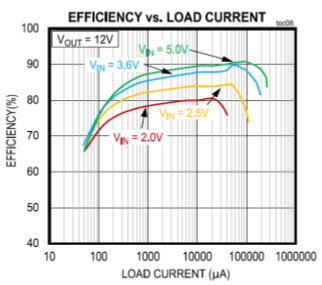Figure 1. MAX17291 efficiency vs. load current (VOUT = 12V).

### Application CircuitFigure 2. 12V Boost converter using MAX17291.

### Operation of MAX17291 Boost Converter IC

A boost converter circuit for 1.8V to 5.5V input and 12V output voltage using MAX17291 IC is shown in Figure 2. The MAX17291 boost converter has two modes of operation, light load efficiency and pulse-width-modulated (PWM). In light load mode, it operates in pulse frequency modulation (PFM) to improve efficiency at light loads. In this mode, the on-time is determined by a peak inductor current limit of 500mA. Once the inductor current hits its limit, the on-time is terminated, and the power diode is forward-biased. In PWM mode, the converter uses a quasi-constant 1.0MHz switching frequency pulse width modulation (PWM) at load current levels in continuous-conduction mode (CCM). Based on the input voltage to output voltage ratio, the circuit predicts the required off-time. In CCM mode, the duty of the boost converter is given by the following equation.### Negative Voltage Using Generic Boost Converter

The circuit to generate negative voltage using a generic boost converter is displayed in Figure 3. As shown in the figure, a charge pump circuit is used from the switching node of the boost converter to generate required negative voltage.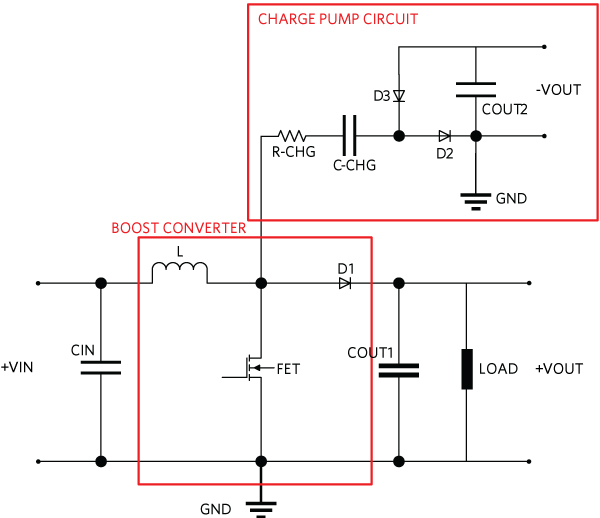Figure 3. Schematic of the negative voltage using the boost converter.

### Operation of Negative Voltage Circuit

For the above circuit, the feedback is taken from output of the boost converter to keep negative output voltage constant. In steady state condition, when the switch Q1 is OFF, the inductor voltage is reversed, and the voltage across Q1 FET is equal to input voltage and inductor voltage. The voltage across Q1 switch is determined by the following equation.

VQ1 = VIN + VL = VOUT + VD1

The current starts flowing from inductor to COUT1 via diode D1 and from inductor to C-CHG via R-CHG and diode D2. The capacitors, COUT1 and C_CHG, are charged during this time. The voltage across the C-CHG is given by the following equation.

VOUT + VD1 = VR-CHG + VC-CHG
VC-CHG = VOUT + VD1 - VRCHG - VD2

The current flow during Q1 OFF state is shown in Figure 4.Figure 4. Current flow during Q1 OFF time.

At the end of the Q1 OFF time, when the Q1 becomes ON, the drain terminal of Q1 is pulled almost to ground. The diode D2 becomes reverse biased and diode D3 becomes forward biased. Once diode D3 becomes forward biased, the current starts flowing from the COUT2 capacitor via R-CHG and diode D3. This appears to lead to a negative voltage on the capacitor COUT2 with respect to ground.

The voltage across COUT2 is given by the following equation.

-VCCHG + VRCHG = VCOUT2 - VD3
VCOUT2 = -VCCHG + VRCHG + VD3

The current flow during Q1 ON state is shown in Figure 5.Figure 5. Current flow during Q1 ON time.

The R-CHG resistor is connected to limit the peak current through the MOSFET Q1 and diodes D2 and D3. Choose between 1? to 2.2? for the value of the R-CHG resistance. If the R-CHG resistor value is too high, the voltage across the R-CHG increases at higher loads, which decreases the voltage across C-CHG & COUT2. If the R-CHG resistor value is too low, then the negative voltage increases at lower load conditions, as there is no sensing of the negative voltage to control.

### Design Specification

• Input: 2.5V to 5.5V
• Output voltage: -11V

### Benefits of Using MAX17291 IC

• Smaller solution size with internal boost MOSFET and diode (1.27mm x 0.87mm WLP package)
• Wide range of negative output voltage achievable
• Can operate from lower input voltage of 1.8V

The MAX17291 boost converter IC has an internal boost MOSFET, diode, and control circuit. A charge pump circuit is connected to the LX switching node of MAX17291 IC to generate a negative voltage. The schematic of negative voltage using MAX17291 IC is shown in Figure 6.Hi-res Image ›
Figure 6. Negative voltage generating using MAX17291 IC.

The required negative output voltage is achieved by setting the feedback network circuit on the output voltage of the boost. Hence, the load regulation performance in the Figure 6 schematic, when compared to the normal positive voltage, is slightly loose because of the charge coupling through the capacitor C-CHG. The switching operation of MAX17291 is based on input voltage and the output voltage of the boost converter. A steady output voltage must be maintained throughout the operation. This is done using a dummy resistor across the OUT pin of the IC and ground. This will act as a dummy load to maintain the positive output voltage constant, which may increase the regulation of negative voltage to some extent.

The load regulation and efficiency performance of the circuit is shown in the graphs below.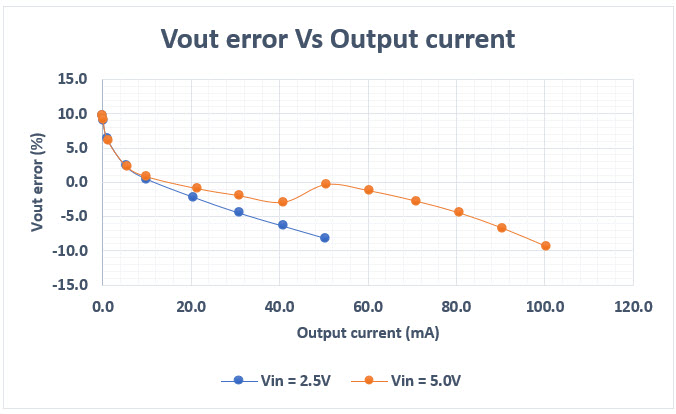Figure 7. Load regulation vs. Output current.Figure 8. Efficiency vs. Output current.

### Load Transient Performance at 2.5Vin & -11Vout ConditionFigure 9. Load transient performance without op-amp.

Condition: VIN = 2.5V, VOUT = -11V, IOUT = 5mA to 30mA

Due to load transient, the voltage change is approximately ~392mV (3.56%). Output voltage is offset by -10V in the waveform.

Waveform: Yellow = VIN, Blue = VOUT, Pink = V , Green = IOUTFigure 10. Load transient performance without op-amp.

Condition: VIN = 2.5V, VOUT = -11V, IOUT = 30mA to 5mA

Due to load transient, the voltage change is approximately ~370mV (3.36%). Output voltage is offset by -10V in the waveform.

Waveform: Yellow = VIN, Blue = VOUT, Pink = VEN, Green = IOUT

### Improving Load Regulation and Transient Performance of a Negative Voltage Circuit

The negative voltage load regulation and transient performances can be improved upon from the previous circuit by directly sensing the negative output voltage. The feedback is then sent to the IC using Maxim's MAX44244AUK+ op-amp for sensing the negative voltage. The output of the op-amp is used to drive the FB pin, which regulates the negative output voltage against load and line transients. The following is a schematic of negative voltage feedback using op-amp.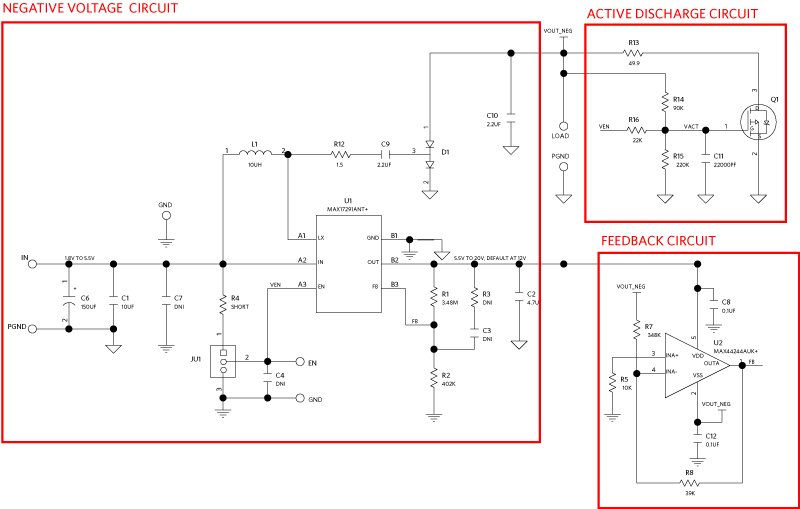Hi-res Image ›
Figure 11. Negative voltage feedback using MAX17291 IC with op-amp.

The following are representations of load regulation and transient performance of negative voltage using an op-amp circuit.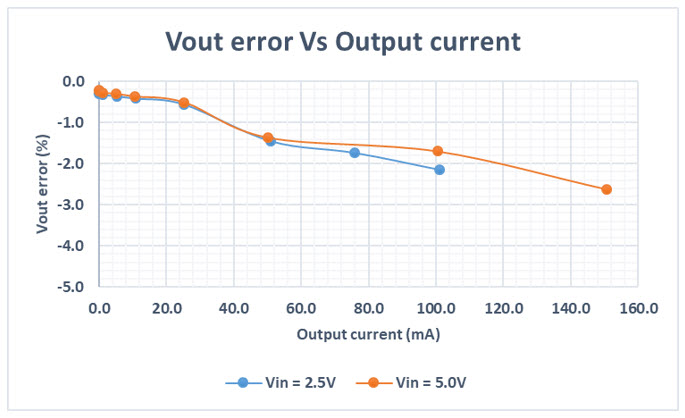Figure 12. Load regulation vs. Output current using an op-amp circuit.Figure 13. Efficiency vs. Output current using an op-amp circuit.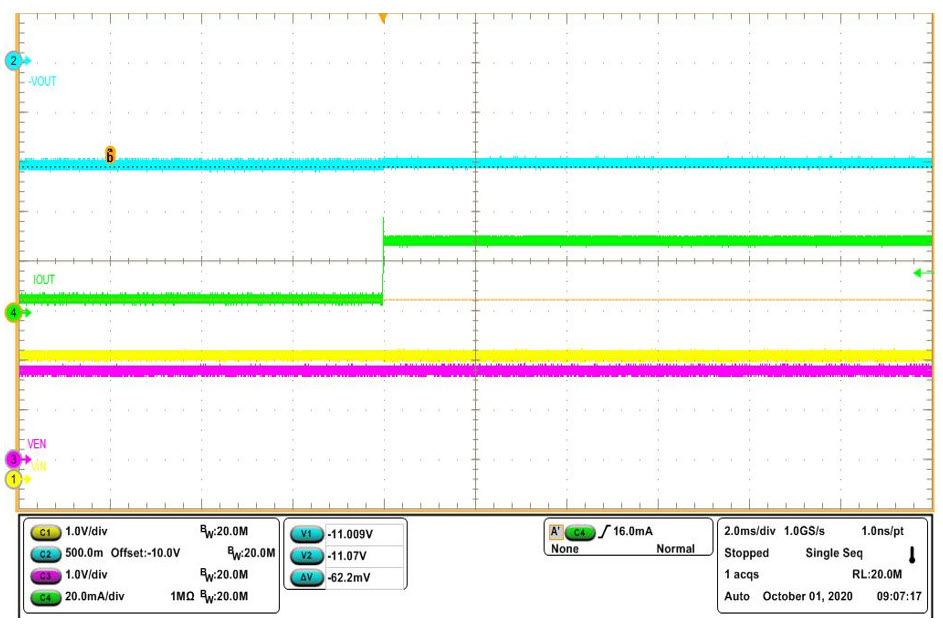Figure 14. Load transient performance using an op-amp circuit.

Condition: VIN = 2.5V, VOUT = -11V, IOUT = 5mA to 30mA

The voltage change due to load transient is around ~62mV (0.56%). Output voltage is offset by -10V in the waveform.

Waveform: Yellow = VIN, Blue = VOUT, Pink = VEN, Green = IOUTFigure 15. Load transient performance using an op-amp circuit.

Condition: VIN = 2.5V, VOUT = -11V, IOUT = 30mA to 5mA

The voltage change due to load transient is around ~72mV (0.65%). Output voltage is offset by -10V in the waveform.

Waveform: Yellow = VIN, Blue = VOUT, Pink = VEN, Green = IOUT

As shown in Figures 7–15, the negative voltage circuit using op-amp has very tight regulation and good transient performance compared to a circuit without op-amp.

### Performance Comparison

Based on the above results, the negative voltage solution with op-amp has good load regulation and efficiency performance.Figure 16. Load regulation performance comparison.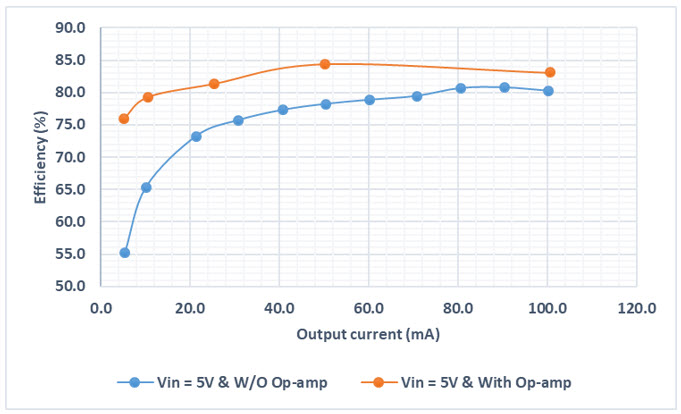Figure 17. Efficiency performance comparison.

### Implementation of Active Discharge Feature

In Figure 11, the active discharge circuit is composed of resistors R13 to R16, capacitor C11, and P-MOS Q1. When the IC is disabled using the EN pin (EN = 0V), the resistors R15 and R16 become parallel, and the voltage Vgs of Q1 is more than the threshold voltage. Hence, the MOSFET Q1 turns ON. The active discharge feature pulls the whole charge, which is accumulated by the COUT2 capacitor, and dumps into the resistor R13. The negative voltage is immediately reduced to a lesser value. When the IC is enabled, the voltage across gate and source is less than the threshold value, and MOSFET Q1 turns OFF. The value of discharge resistor can be chosen based on the required discharge time of the COUT2 capacitor.

The operation of active discharge is shown in the following waveform.Figure 18. Active discharge feature.

### Conclusion

Negative output voltage is generated from a positive input voltage using MAX17291 IC. Users can improve the load regulation and transient performance of the negative output voltage by adding the op-amp circuit and implementing the active discharge feature to the negative output voltage circuit.